November 30, 2023

Remember Me

### Create an account

Fields marked with an asterisk (*) are required.
Name *
Email *
Verify email *## Association Rules

Machine Learning

User Rating: 5 / 5## SVM

Machine Learning

User Rating: 0 / 5## I. Large margin classification:

a. Optimization objective:

There's one more algorithm that is very powerful and is very widely used both within industry and academia, and that's called the support vector machine. And compared to both logistic regression and neural networks, the Support Vector Machine, or SVM sometimes gives a cleaner, and sometimes more powerful way of learning complex non-linear functions.

## Naïve Bayes

Machine Learning

User Rating: 0 / 5# Naïve Bayes

## Definition:

• Naïve Bayes overview:
• Relationships between input features and class expressed as probabilities.
• Label for sample is class with highest probability given input.
• Naïve Bayes classifier:
• Classification using probability
• Bayes theorem: it makes estimating the probabilities easier.
• Feature independence assumption: For a given class, the value of one feature does not affect the value of any other feature.
• The naïve independence assumption and the use of Bayes theorem gives this classification model its name.
• Probability of event:
• Probability is measure of how likely an event is
• Probability of event ‘A’ occurring:• Joint probability:
• Probability of events A and B occurring together: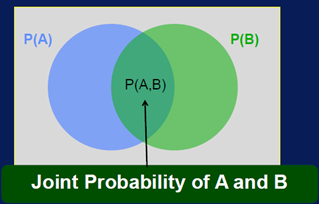• If the 2 events are independent: P(A, B) = P(A) * P(B)
• Conditional probability:
1. Probability of event A occurring, given that event B occurred.
2. Event A is conditioned on event B. P(A|B) = P(A,B)/P(B)

It provides the means to specify the probability of a class label, given the input values.

• Bayes’ theorem:
• Relationship between P(B|A) and P(A|B) can be expressed through Bayes’ theorem:• Classification with probabilities:

Given features X = {X1, X2,……,Xn}, predict class C. Do this by finding value of C that maximizes P(C|X)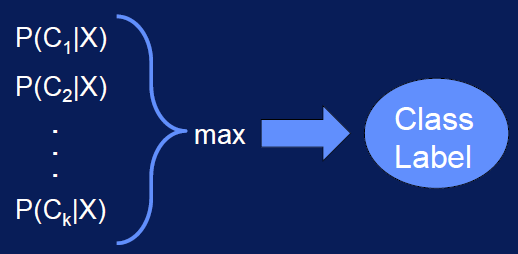• Bayes theorem for classification:
• But estimating P(C|X) is difficult, we should use Bayes’ theorem to simplifies the problem:• So to get P(C|X), only need to find P(X|C) and P(C).
• Estimating P(C): To estimate P(C), calculate fraction of samples for class C in training data.
• Estimating P(X|C):
• Independence assumption: Features are independent of one another:

P (X1, X2,…Xn|C) = P(X1|C) * P(X2|C) * …….. * P(Xn|C)

• To estimate P(X|C), only need to estimate P(Xi|C) individually

• Naïve Bayes classification:
• Fast and simple: the probabilities that are needed can be calculated with a single scan of the data set and stored in a table.
• Scales well:
• Model building and testing of both task, it scales well.
• Due to the independent assumption:
1. The probability for each feature can be independently estimated.
2. Featured probability is can be calculated in very low.
3. The data set size does not have to grow exponentially with a number of features.
4. This avoid the many problems associated with the curse of dimensionality.
5. No need to a lot of data to build the model.
6. Number of parameters scales linearly with the number of features.

• The independence assumption may not hold true
• In practice, still works quite well.
• Does not model interactions between features.

author: LASSRI Safae
PhD at faculty of science Ben M'Sik.

## Logistic Regression

Machine Learning

User Rating: 0 / 5## Logistic Regression: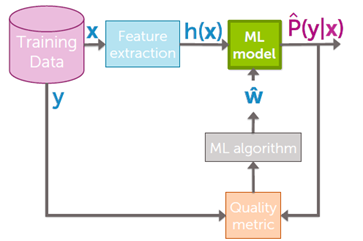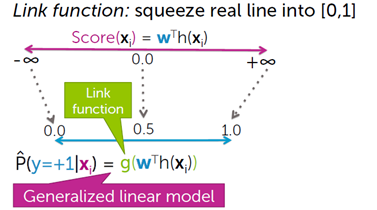Scores range from minus infinity to plus infinity, probabilities range between 0 and 1. The question is how do we relate score from minus infinity to plus infinity, to probability 0 and 1. How do we link these two things?

We are going to take the score, which is between minus infinity and plus infinity, we are going to push it through a function g that squeezes that huge line into the interval 0, 1. And uses it to predict the probability that y equals +1.

And when you're taking a linear model, w transpose h minus infinity to plus infinity and you're squeezing it into 0,1 using link functions you are building what's called a generalized linear model.

what's a generalized linear model?

It's just like a regression model, but you squeeze it into 0, 1 by pushing through a link function.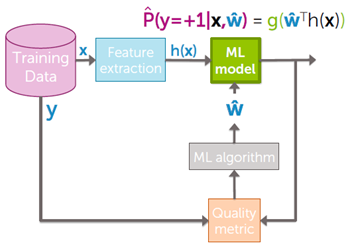The sigmoid (or logistic) link function:a. Classification and representation:

i. Classification:

To attempt classification, one method is to use linear regression and map all predictions greater than 0.5 as a 1 and all less than 0.5 as a 0. However, this method doesn't work well because classification is not actually a linear function.

The classification problem is just like the regression problem, except that the values y we now want to predict take on only a small number of discrete values. For now, we will focus on the binary classification problem in which y can take on only two values, 0 and 1. (Most of what we say here will also generalize to the multiple-class case.) For instance, if we are trying to build a spam classifier for email, x(i) then   may be some features of a piece of email, and y may be 1 if it is a piece of spam mail, and 0 otherwise. Hence, y∈ {0,1}. 0 is also called the negative class, and 1 the positive class, and they are sometimes also denoted by the symbols “-” and “+.” Given x(i), the corresponding y(i) is also called the label for the training example.

ii. Hypotheses representation:

We could approach the classification problem ignoring the fact that y is discrete-valued, and use our old linear regression algorithm to try to predict y given x. However, it is easy to construct examples where this method performs very poorly. Intuitively, it also doesn’t make sense for hθ(x) to take values larger than 1 or smaller than 0 when we know that y ∈ {0, 1}. To fix this, let’s change the form for our hypotheses hθ(x) to satisfy 0≤hθ(x)≤1. This is accomplished by plugging θTx  into the Logistic Function.

Our new form uses the "Sigmoid Function," also called the "Logistic Function":The following image shows us what the sigmoid function looks like: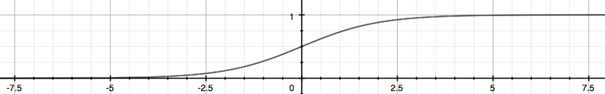The function g(z), shown here, maps any real number to the (0, 1) interval, making it useful for transforming an arbitrary-valued function into a function better suited for classification.

hθ(x) will give us the probability that our output is 1. For example, hθ(x)= 0.7 gives us a probability of 70% that our output is 1. Our probability that our prediction is 0 is just the complement of our probability that it is 1 (e.g. if probability that it is 1 is 70%, then the probability that it is 0 is 30%).iii. Decision Boundary:

In order to get our discrete 0 or 1 classification, we can translate the output of the hypothesis function as follows:The way our logistic function g behaves is that when its input is greater than or equal to zero, its output is greater than or equal to 0.5:Remember:So, if our input to g is x, then that means:From these statements we can now say:The decision boundary is the line that separates the area where y = 0 and where y = 1. It is created by our hypothesis function.

Example:In this case, our decision boundary is a straight vertical line placed on the graph where x1 = 5, and everything to the left of that denotes y = 1, while everything to the right denotes y = 0.

Again, the input to the sigmoid function g(z) (e.g. θTx) doesn't need to be linear and could be a function that describes a circle (e.g. z=θ01x122x22) or any shape to fit our data.

b. Logistic regression model:

i. Cost Function:

We cannot use the same cost function that we use for linear regression because the Logistic Function will cause the output to be wavy, causing many local optima. In other words, it will not be a convex function.

Instead, our cost function for logistic regression looks like:When y = 1, we get the following plot for J(θ) vs hθ(x):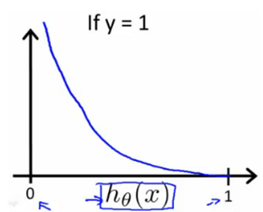Similarly, when y = 0, we get the following plot for J(θ) vs hθ(x):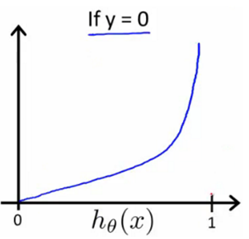If our correct answer 'y' is 0, then the cost function will be 0 if our hypothesis function also outputs 0. If our hypothesis approaches 1, then the cost function will approach infinity.

If our correct answer 'y' is 1, then the cost function will be 0 if our hypothesis function outputs 1. If our hypothesis approaches 0, then the cost function will approach infinity.

Note that writing the cost function in this way guarantees that J(θ) is convex for logistic regression.

ii. Simplified Cost Function and Gradient Descent:

We can compress our cost function's two conditional cases into one case:Notice that when y is equal to 1, then the second term (1-y)log(1-hθ(x)) will be zero and will not affect the result. If y is equal to 0, then the first term -ylog(hθ(x))will be zero and will not affect the result.

We can fully write out our entire cost function as follows:A vectorized implementation is:Remember that the general form of gradient descent is:

Repeat {}

We can work out the derivative part using calculus to get:

Repeat {}

Notice that this algorithm is identical to the one we used in linear regression. We still have to simultaneously update all values in theta.

A vectorized implementation is:"Conjugate gradient", "BFGS", and "L-BFGS" are more sophisticated, faster ways to optimize θ that can be used instead of gradient descent. We suggest that you should not write these more sophisticated algorithms yourself (unless you are an expert in numerical computing) but use the libraries instead, as they're already tested and highly optimized. Octave provides them.

We first need to provide a function that evaluates the following two functions for a given input value θ:We can write a single function that returns both of these: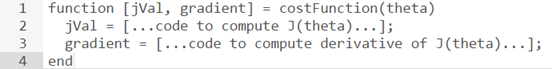Then we can use octave's "fminunc()" optimization algorithm along with the "optimset()" function that creates an object containing the options we want to send to "fminunc()". (Note: the value for MaxIter should be an integer, not a character string - errata in the video at 7:30)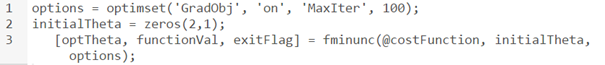We give to the function "fminunc()" our cost function, our initial vector of theta values, and the "options" object that we created beforehand.

c. Multiclass classification:

i. Multiclass Classification: One-vs-all :

Now we will approach the classification of data when we have more than two categories. Instead of y = {0,1} we will expand our definition so that y = {0,1...n}.

Since y = {0,1...n}, we divide our problem into n+1 (+1 because the index starts at 0) binary classification problems; in each one, we predict the probability that 'y' is a member of one of our classes.We are basically choosing one class and then lumping all the others into a single second class. We do this repeatedly, applying binary logistic regression to each case, and then use the hypothesis that returned the highest value as our prediction.

The following image shows how one could classify 3 classes: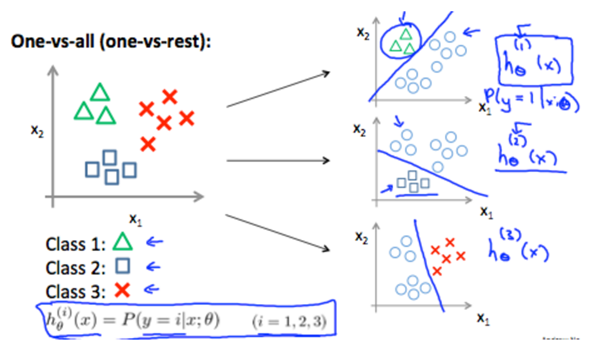To summarize:

Train a logistic regression classifier hθ(x) for each class to predict the probability that y = i.

To make a prediction on a new x, pick the class that maximizes hθ(x).

auhor: LASSRI Safae
PhD at faculty of science Ben M'Sik.

## LINEAR CLASSIFIER

Machine Learning

User Rating: 0 / 5## 1- Linear classifier: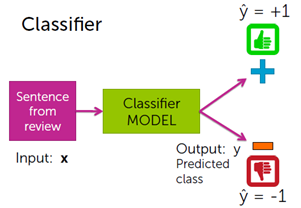A linear classifier:

• Using a training data to learn a weight or coefficient for each word.
• Calling a linear classifier, because output is weighted sum of input.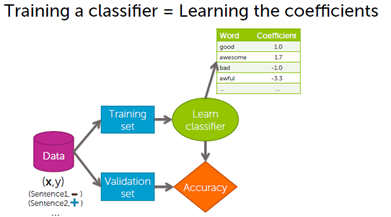Decision boundries:

Decision boundries separates positive and negative predictions:

• For linear classifiers:
• When 2 coefficients are non-zero Line
• When 3 coefficients are non-zero Plane
• When many coefficients are non-zero Hyper plane
• For more general classifiers More complicated shapes.

Linear classifier model: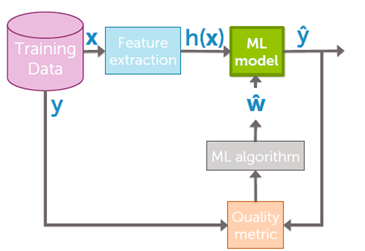a- Linear regression with one variable:

i- Model and cost function:

1-Model representation:

Our first learning algorithm will be linear regression.

More formally, in supervised learning, we have a data set and this data set is called a training set.

Let's define some notation that we're using throughout this course. We're going to define quite a lot of

symbols.

• m: to denote the number of training examples.
• x: to denote the input variables often also called the features.
• y: to denote my output variables or the target variable which i'm going to predict.
• (x,y): to denote a single training example.

To establish notation for future use, we’ll use  to denote the “input” variables (living area in this example), also called input features, and  to denote the “output” or target variable that we are trying to predict (price). A pair   is called a training example, and the dataset that we’ll be using to learn—a list of m training examples  —is called a training set. Note that the superscript “(i)” in the notation is simply an index into the training set and has nothing to do with exponentiation. We will also use X to denote the space of input values, and Y to denote the space of output values. In this example, X = Y = ℝ.

To describe the supervised learning problem slightly more formally, our goal is, given a training set, to learn a function h: X → Y so that h(x) is a “good” predictor for the corresponding value of y. For historical reasons, this function h is called a hypothesis. Seen pictorially, the process is therefore like this: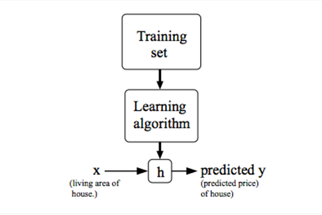When the target variable that we’re trying to predict is continuous, such as in our housing example, we call the learning problem a regression problem. When y can take on only a small number of discrete values (such as if, given the living area, we wanted to predict if a dwelling is a house or an apartment, say), we call it a classification problem.

2-Cost function:

We can measure the accuracy of our hypothesis function by using a cost function. This takes an inputs from x's and the actual output y's.To break it apart, it is  where   is the mean of the squares of   , or the difference between the predicted value and the actual value.

This function is otherwise called the "Squared error function", or "Mean squared error". The mean is halved  as a convenience for the computation of the gradient descent, as the derivative term of the square function will cancel out the.

3-Cost function - Intuition 1

If we try to think of it in visual terms, our training data set is scattered on the x-y plane. We are trying to make a straight line (defined by ) which passes through these scattered data points.

Our objective is to get the best possible line. The best possible line will be such so that the average squared vertical distances of the scattered points from the line will be the least. Ideally, the line should pass through all the points of our training data set. In such a case, the value of  will be 0. The following example shows the ideal situation where we have a cost function of 0.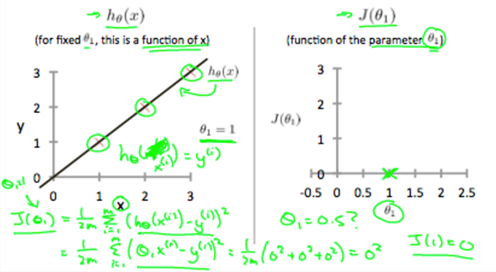When θ1=1, we get a slope of 1 which goes through every single data point in our model. Conversely, when θ1=0.5, we see the vertical distance from our fit to the data points increase.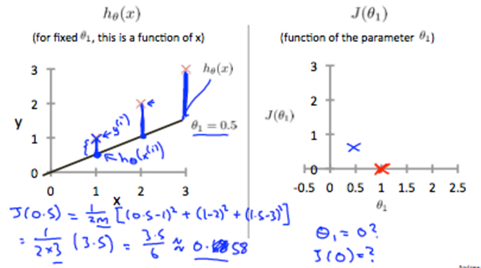This increases our cost function to 0.58. Plotting several other points yields to the following graph:

Thus as a goal, we should try to minimize the cost function. In this case, =1 is our global minimum.

ii- Parameter learning: